# IP Address Classes: the Definitive Guide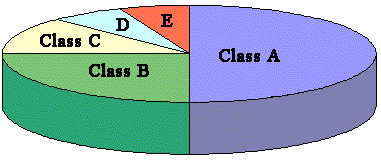Ever wondered how the IP Address Classes are defined and used in the network devices? If so, keep reading!

Electronic devices such as computers and routers rely on an internal binary architecture. They use bits that can only have two mathematical values: ‘0’ and ‘1’. The decimal/binary conversion is fundamental to understand how the network addressing and subnetting operate.

The IP Address is represented by a 32 bits binary number such as:

11000000.10101000.00000001.00000001

A long string of bits is not easy to use for a human being, so to make the utilisation easier this number is normally broken into octets that are groups of 8 bits each (as illustrated by the dots inserted into the above binary string). The IP address of 32 bits is partitioned in 4 octets, so each of them can be converted to decimal notation.

First Octet Second Octet Third Octet Fourth Octet
11000000 10101000 00000001 00000001
192 168 1 1

The binary and decimal notations are two different ways of representing the same number, and so the same IP address:

11000000.10101000.00000001.00000001 = 192.168.1.1

The decimal notation 192.168.1.1 is more intuitive and always used for the manual configurations of a network device. This latter will take care of converting it in binary for the use in the internal software and electronic circuits.

Before beginning with the address class explanation, it’s important clarify the terminology that will be used from now on. If some of these definitions sound a bit unclear, please keep reading as everything should be explained by the end of this post!

• IP Address: a unique 32 bits number assigned to any device connected to a network. Devices such as laptops (also called hosts) usually have only one connected interface (e.g. ethernet or wireless) while more complex equipments like routers could have several interfaces connected to different networks. Each of them require a unique IP address assigned.
• Network Part: portion of the IP address that determines the subnet.
• Host Part: portion of the IP address that identifies the host within the subnet.
• Subnet: network obtained using a portion of a bigger network.
• Subnet Mask: bit mask used to understand which part of the IP address is the Network Part and which one is the Host Part.
• Interface: a connection to the network, it can belong to a host or to a router.

The IP addresses are divided in 5 classes, each one identified by a letter:

Class Range First Octet Network/
Host
Number of Network bits Number of Host bits
A 1-127 N.h.h.h 8 24
B 128-191 N.N.h.h 16 16
C 192-223 N.N.N.h 24 8
D 224-239 not to be used for hosts
E 240-255 not to be used for hosts

The class is determined by the value of the first octet!

Some IP addresses are reserved for specific uses, for example 127.0.0.1 is always utilised as localhost (RFC-3330). Another example is 0.0.0.0 used to indicate the network to which the device is currently connected.

Using the table presented above, let’s see some examples of class identification:

2.67.9.67 –> First Octet in the range 1-127 –> Class A

147.34.21.88 –> First Octet in the range 128-191 –> Class B

192.168.1.1 –> First Octet in the range 192-223 –> Class C

224.1.1.1 –> First Octet in the range 224-239 –> Class D

250.8.67.54 –> First Octet in the range 240-255 –> Class E

The classes A, B, C, D are used in the actual networks, but not the class E that is reserved and then not available for the data routing. From the subnetting perspective that does not matter, since the whole IP addressing space follows the same mathematical rules.

To visualize how the entire addressing space is partioned, we can refer to the following image: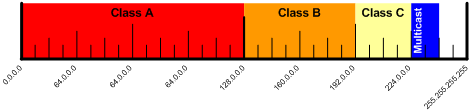The Class A addresses occupy half of the addressing space. The Class B is taking half of the remaining space and so on for the Class C and Class D (Multicast). The left over is assigned to the Class E for experimental purposes, but never used for the data traffic routing.

Each address class has a default Subnet Mask that is not explicitly specified, as shown in the following table. The meaning of the subnet mask is explained here. In the Default Subnet Mask column are indicated different notations to represent the same number.

Class Range First Octet Network/Host Number of Network bits Number of Host bits Default Subnet Mask
A 1-127 N.h.h.h 8 24 /8
255.0.0.0
B 128-191 N.N.h.h 16 16 /16
255.255.0.0
C 192-223 N.N.N.h 24 8 /24
255.255.255.0
D 224-239 not to be used for hosts
E 240-255 not to be used for hosts

The IP addresses that use default subnet masks are called classful. In these addresses the subnet can only be one of the types included in the previous table:

• /8 for Class A
• /16 for Class B
• /24 for Class C

The following image explains how the 32 bits of the IP address are partitioned depending on the class: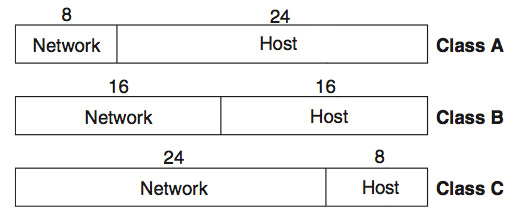In Class A the first octet (8 bits so subnet /8) is used for the network part, while the remaining three octets (24 bits) for the host part. In Class B the first two octets (16 bits so subnet mask /16) are used for the network part and the second two (16 bits) for the host part. In Class C the first three octets (24 bits so subnet mask /24) are for the network part, while the last one (8 bits) for the host part. Depending on the class the number of available bits for network/host parts changes, and so the number of networks/hosts available as summarised in the following table:

Class First Octet bits Network/Host Number of Network bits Number of Host bits Number of Networks e Hosts available
A 0xxx xxxx N.h.h.h 8 24 126 networks (2^7 – 2)
16.777.214 hosts per network (2^24 -2)
B 10xx xxxx N.N.h.h 16 16 16.384 networks (2^14)
65.534 hosts per network (2^16- 2)
C 110x xxxx N.N.N.h 24 8 2.097.152 networks (2^21)
254 hosts per network (2^8 -2)
D 1110 xxxx not to be used for hosts
E 1111 xxxx not to be used for hosts

In the calculation of the maximum number of hosts per network, the subtraction of two addresses ‘-2’ shown in the table is due to the base address of the subnet and the broadcast address that are both not valid IP to use with the hosts.

In the calculation of the maximum number of networks, it’s necessary to keep in mind the number of fixed bits in the first octet. As illustrated in the table above, depending on the class there are bits that can’t change in the first octet. There is one fixed bit in Class A (0xxxxxxx), two fixed bits in Class B (10xxxxxx) and three fixed bits in Class C (110xxxxx). These fixed bits need to be subtracted before calculating the power of two, and that’s why in Class A we have 2^7 (i.e. 8-1=7), in Class B 2^14 (i.e. 16-2=14) and in Class C 2^21 (i.e. 24-3=21). The fixed bits are used to recognise the class of an IP address, as defined in the IP Protocol specifications (RFC-791).

The mechanisms of Classful IP addresses was designed along with the IP Protocol, when the number of existing computers was still very limited. The basic idea was allocating Class A addresses to large companies where a significant number of internal hosts needed to be connected. The Class B was planned for medium-size enterprises while the Class C for small businesses. Class D was reserved for Multicast, a streaming technology to use with applications such as real time video. Class E has always been reserved for experimental use so it’s not possible to use for packet routing.

Examples of long time allocation of IP addresses are: block of addresses 9.0.0.0/8 allocated to IBM, block 13.0.0.0/8 to Xerox, block 17.0.0.0/8 to Apple. For an exhaustive list please refer to this page.

With the growing demand of IP addresses, it became clear that having just three classes of IP was causing a large wasting of addressing space.

In order to have a more efficient use of the addressing space, the IP Classless mechanism was designed. It relies on the CIDR/VLSM mechanism. A new field called Subnet was introduced in the IP address. The subnet is placed between the network part and the host part, “stealing” bits to this latter. The network part is never reduced, while the host part decreases depending on the number of “stolen” bits. The concept is pretty easy to explain using a picture, where we can see that the subnet part has variable length while the host part needs to shrink accordingly: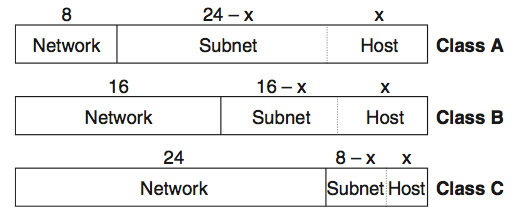Having introduced the Subnet field, now the subnet mask can have different values than the classic defaults /8, /16, /24. For example the following IP address is perfectly valid using the classless mechanism:

72.2.2.2 /26

This is a Class A address being the first octet in the range 1-127, so the network part occupies 8 bits. The remaining 24 bits will be partitioned between the subnet part (using 18 bits) and the host part (using 6 bits). Here is the math to find these values:

(IP address length) – (subnet mask length) = 32 – 26 = 6 bits for the host part

(subnet mask length) – (network part length) = 26 – 8 = 18 bits for the subnet part

We can graphically represent the IP address as follows: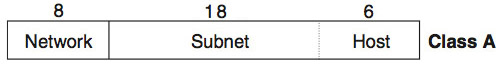In summary, the subnet mask defines the number of bits assigned to the Network+Subnet part, while the remaining bits are left to the host part. To explain this fundamental concept let’s use an example. The IP address that we use is a typical Class C with default subnet mask /24. Also the default subnet masks can be used in the classless notation:

200.1.1.1 255.255.255.0 or /24

As shown in the table, the subnet mask notation is similar to the IP address one, but the meaning is very different. To understand how to use the subnet mask, we need to convert it in binary:

255.255.255.0  =  11111111.1111111.11111111.00000000

## Subnet mask: how does it work?

The subnet mask is always formed by a string of  ‘1’ that starts from the left side and continues without interruptions. The part composed by ‘1’ indicates the Network+Subnet part of the associated IP address, while the ‘0’ the host part. In this specific case there will not be any subnet because we are using a default subnet mask /24, and this was done on purpose to start with an easy example.

Executing a bitwise AND between the IP address and the Subnet Mask the result in binary will be:

IP Address: 200.1.1.1 11001000 00000001 00000001 00000001
AND AND AND AND
Subnet Mask: 255.255.255.0 11111111 11111111 11111111 00000000
= = = =
Subnet 11001000 00000001 00000001 00000000

Converting now the subnet in decimal:

11001000.00000001.00000001.00000000 = 200.1.1.0

In summary:

Subnet Network 200.1.1.0

What was just illustrated is the subnet network calculation for a specific IP address. the calculation was done exactly how it happens in the electronic devices which make use of binary operations such as the bitwise AND.

If you are not familiar on how to calculate the IP subnetting, you might want to have a look at our Easy Subnetting Technique that allows to solve any subnetting problem in less than a minute.

## Conclusion

In this article we saw how the IP Address Classes are defined and used in the network devices. We also illustrated the differences between Classful and Classless IPs, and how a variable length subnet mask helps in optimising the use of the IP addressing space, still keeping the addresses classified as A, B, C and D.#### Daniele Besana

Daniele is a freelancer consultant with 15 years of experience in network security, customer support, Linux and Salsa. He worked for Juniper Networks in Netherlands, providing support and consultancy on security projects across Europe and Middle-East.

1.I understand how to subnet and calculate the number of hosts available for a said subnet. However, I struggle with calculating how many subnets are available for a given subnet. for example, The number of subnets available changes based on the class of IP address. Is there a given formula out there to calculate the number of subnets?
Thanks

2.localhost is 127.0.0.1 ( not 127.0.0.0 )

•ups..! Just fixed, thanks! 🙂

This site uses Akismet to reduce spam. Learn how your comment data is processed.